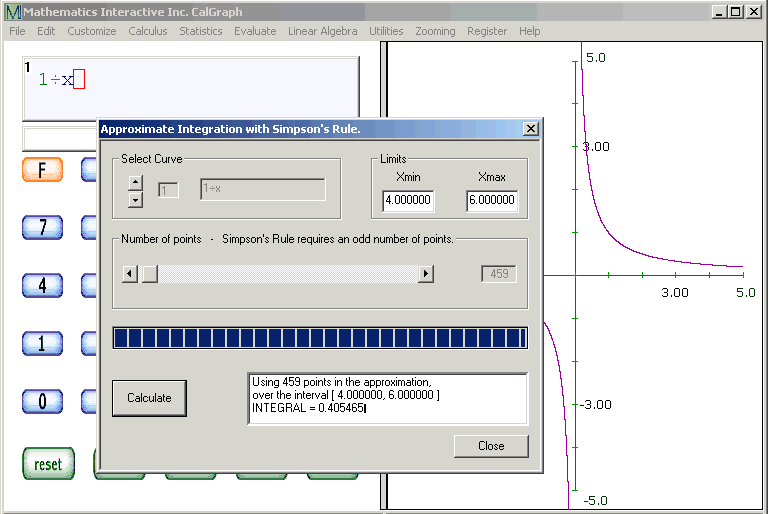# Integrate

CalGraph will calculate the area under a curve, what is called the definite integral.

Enter the function and then click Calculus and Approximate Integration. You get a window like this.If you have several curves in CalGraph, use thearrows to select which one you want to integrate.

The formula for the curve appears in the second grey box.

A definite integral runs between two values of x. Enter the lower value in the Xmin box and the higher one in the Xmax box.

Slide the button alongto tell CalGraph how many points to use. More points takes a little bit longer to calculate, but is more accurate.

The example shows thatusing Simpson’s Rule with 459 points.

The Xmin should be lower than the Xmax. If it is not, the integral will be negative.

If the curve is below the x-axis, the curve is negative, so the integral is negative.  Be sure this is what you meant.

If a function goes undefined, the area does not make sense, so you get the error message INPUT ERROR OR DISCONTINUITY. Sometimes Simpson's Rule will give a number answer when it should give this error.